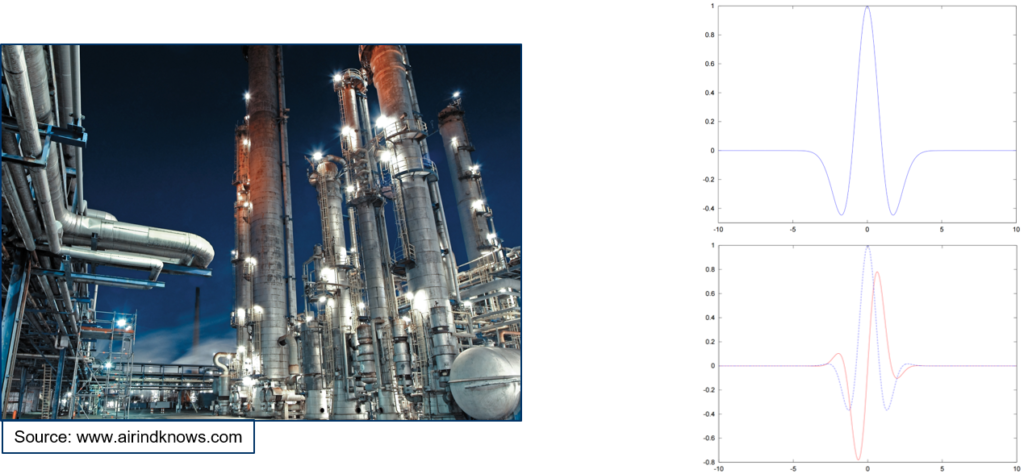# Adaptive Wavelet Methods for Control of Networks## Adaptive Wavelet Methods for Control of Networks

Research Activities:

1.)  Method development for wavelet-based numerical methods for optimal control of quasilinear hyperbolic balance laws on networks;

2.)  Viscosity solutions for hyperbolic systems on networks;

Transport processes in networked systems play a fundamental role in energy networks, structural mechanics, fluid mechanics, medicine and traffic networks. Typically, we have an infrastructure or a physical environment, such as a network of pipes, arteries, open channels, transmission lines, and networks for public transport. The physical process running in such an environment has to be considered, such as the flow of gas, water, blood, traffic, and currents. When it comes to real-world applications, such networks may consist of thousands of elements (pipes, beams, roads etc.). Because these physical processes are a type of propagation, they are typically modeled by hyperbolic equations or systems, and sometimes with an inherent viscosity. Consequently, the network problem appears to be a coupled system of equations: One set of equations for the process, and another set for the infrastructure that it carries. The networks of infrastructures can be adequately described mathematically by hyperbolic equations of second order in space and time, while the processes are often represented by systems of quasilinear hyperbolic balance laws (such as the 1-D Euler gas system with nonlinear friction terms).

The main goal is to not only describe and observe the dynamic behavior of such distributed transport networks but rather to exert controls to influence the performance according to measurements and control objectives (often called cost functions). For large networks, this is a formidable task. Besides the control-theoretic investigations, an effective, adaptive, and in particular multiscale numerical simulation method will be applied. The classical approach to numerical simulation of the described transportation networks is the finite volume method. However, in recent investigations flow problems are addressed using wavelets. Wavelets, as the origin of multi-resolution analysis, are prone to model reduction, compression, and multi-scale analysis.

With this research project, we intend to pursue adaptive wavelet methods for quasilinear hyperbolic systems on networks. We will work on theoretical aspects of Wavelet-Galerkin approximations in the context of controllability, stabilizability, as well as optimal control. The project is likely to succeed with methods similar to moment theory that have been developed earlier. These methods depend on the Eigen elements of the underlying differential operators. Methods for networks provide especially challenging problems from the multi-resolution point of view. The theoretical will be complemented via the numerical realization of the wavelet-based simulations.

Viscosity solutions for these systems also require wavelet methods on general manifolds, and the fast diffusion wavelet method will be very efficient. Viscosity solutions, on the level of artificial viscosity, provide the smoothing necessary for the smoothness of the control-to-state-maps and is, therefore, the starting point for an adjoint calculus. Since adjoint equations are the result of linearization of the original equations along the optimal trajectory they involve linear systems of the same type. With adaptive wavelet methods, the coupled system (state and adjoint) can be handled using the same method.

We expect a breakthrough in handling multi-scale optimal control problems for flow problems in networks. We further expect that the genuine multi-resolution character of the underlying wavelets will provide us, for the first time, with a genuine scale-bridging paradigm by a single paradigm. Extensions to real-life gas networks will help to validate these methods and provides an important outreach of applied mathematics into the industry. This is particularly important for India, where such potential is often still not considered by decision-makers.